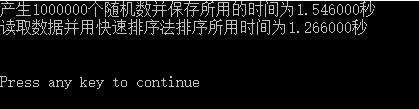# C产生正态分布随机数写入文件并读出后用快速排序法排序

## 基于快速排序法的正态随机数排序

• 使用中心极限定理产生正态分布随机数
• 使用快速排序法进行排序
• 读写数据到记事本
• 程序计时
#include <stdio.h>
#include <stdlib.h>
#include <math.h>
#include <time.h>
#define N 1000000

double norm(double mu,double sigma)
{
double x,stdnorm,sum=0.0;int j;

for(j=1;j<=12;j++)
{sum=sum+rand()/(RAND_MAX+1.0);}
stdnorm=sum-6;
x=sigma*stdnorm+mu;
return x;
}

void QKSORT(int min_index,int max_index)
{
extern double data[N];
if(max_index<=min_index+1&&data[min_index]<=data[max_index])
return;
double model=data[min_index],temp;
int begin_index=min_index,terminal_index=max_index;
if(max_index<=min_index+1&&data[min_index]<=data[max_index])
return;
max_index++;
while(min_index!=max_index)
{
for(max_index=max_index-1;max_index>=min_index;max_index--)
{
if(data[max_index]<model||max_index==min_index)
break;
}
if(max_index==min_index)
break;
for(min_index=min_index+1;min_index<=max_index;min_index++)
{
if(data[min_index]>model||min_index==max_index)
break;
}
if(max_index!=min_index)
{temp=data[min_index];
data[min_index]=data[max_index];
data[max_index]=temp;}
}
data[begin_index]=data[max_index];data[max_index]=model;
if(begin_index<max_index-1)
QKSORT(begin_index,max_index-1);
if(max_index+1<terminal_index)
QKSORT(max_index+1,terminal_index);
}

double data[N];
void main()
{
int i;clock_t sort_start,sort_end,rand_start,rand_end;
void QKSORT(int min_index,int max_index);
double norm(double mu,double sigma);
double data0;
rand_start=clock();
srand(time(0));
FILE *fp;
fp=fopen("D:\\data.txt","w+");
for(i=0;i<=N-1;i++)
{data0=norm(0,1);
fprintf(fp,"%lf\n",data0);
}
fclose(fp);
rand_end=clock();

sort_start=clock();
if((fp=fopen("D:\\data.txt","r"))==NULL)
{
printf("cannot find what you need,please check it!");
exit(0);
}
for(i=0;i<=N-1;i++)
{fscanf(fp,"%lf",&data[i]);
//printf("%lf\t",data[i]);
}
fclose(fp);
QKSORT(0,N-1);
sort_end=clock();

fp=fopen("D:\\data_sorted.txt","w+");
for(i=0;i<=N-1;i++)
fprintf(fp,"%lf\n",data[i]);
fclose(fp);
//for(i=0;i<=N-1;i++)
//  printf("%lf\n",data[i]);
/*不建议打印出来，打印时间较慢,排序前后文件已保存到D盘，请查看*/

printf("产生%d个随机数并保存所用的时间为%lf秒\n读取数据并用快速排序法排序所用时间为%lf秒\n\n\n",N,(double)(rand_end-rand_start)/CLOCKS_PER_SEC,(double)(sort_end-sort_start)/CLOCKS_PER_SEC);

}### 程序说明

1. 定义函数norm用于随机产生一个服从均值为mu，标准差为sigma的正态分布随机数。其中stdnorm为由利用中心极限定理产生的服从标准正态分布的数值，为何使用for循环循环12次等相关细节处理，请自行百度”中心极限定理“。（以下及以后文中提到的细节方面的处理，请自行查询，不再赘述，在此做统一说明。）主函数中的srand和rand配合使用，避免c伪随机数每次产生的随机数数值都一样。
2. 定义第二个函数QKSORT，意指quike sort，表示快速排序法。输入为数值的最小和最大下标，对数组进行从小到大的排序。快速排序法是基于冒泡排序法的一个改进，虽然不是最快的算法，本人认为确实性价比最高的排序方法，值得一学。
3. 快速排序法的主要思想：在一列数中随意取一个数作为标兵，不妨就取第一个数，在剩下的数中，先从右边（数列后定义为右）起，从右到左找到第一个比标兵小的数，记下来。接着从左起，从左到右找到第一个比标兵大的数，也记下来。把找到的两个数交换位置。接着从之前右边找到那个比标兵小的数原来的位置起，从右到左再次开始搜寻比标兵小的数，左边如是，再交换……直到从右边开始搜索和从左边开始搜索碰头了，这时再碰头的位置，左边的数都比标兵小，右边的数都比标兵大，想办法把标兵插到当中去，使得标兵左边的数都比标兵小，右边的数都比标兵大，就算完成了一次排序。接着以标兵为分界点，将一次排序后的数列分成两个部分，对两个部分同样所述方法进行排序，一直进行下去，若干次以后，整个数列就按从小到大的顺序排好了。想法比较简单，但中间有一些细节不好处理，请自行想办法。实在想不出来，请参考我的程序。
4. 快速排序法，用了递归的思想。我写得比较笼统，欲进一步了解，请点击这里
5. 读写文件的格式为：
FILE *fp;
fp=fopen("D:\\data.txt","w+");
fclose(fp);

------------------------------------------------------

FILE *fp;
if((fp=fopen("D:\\data.txt","r"))==NULL)
{
printf("cannot find what you need,please check it!");
exit(0);
}

fprintf(fp,"%lf\n",data);
fclose(fp);
1. 计时要用到头文件time.h，计时的函数有很多，有clock（），time（）等。请自行脑补。

• 注意要用到的头文件，宁可多，不可少。
• 主函数内的动态数组元素个数不能超过130000，申请不到空间，容易出错，注意将数组改写成static或者全局变量。
• 使用printf函数打印将花费不少时间，数据量较大时，建议直接保存到txt文件中。
• 看别人程序是一件蛮痛苦的事情，建议掌握好算法思想后，自己动手写程序，网上有大量资源可供参考。
• vc++6.0除了报错摁F4之外，还要学会调试。

12-1809-192857
06-2020
11-03306
07-16401
02-14746
04-12
12-10904
09-233124
07-23151
03-23419
03-1125
08-18874
01-119万+
12-20
03-28250
10-22636